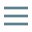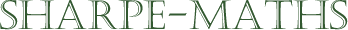Previous topic:
Simple linear equations
Current topic:
Complex linear equations
Next topic:
Simultaneous equations

### Equations with variables on both sides

Once we get to equations of this kind, where we have unknowns on both sides, this is the moment when like St Paul, in 1 Corinthians 13, we must Put away childish things. It is now time to say bye-bye to the flow chart method of solving equations. It doesn't work any more and if you have not started to use a slightly more grown-up, algebraic method, now is your time. I would suggest going back and working through some of the harder questions in the previous section, before embarking on this new material.

### Equations with brackets

Brackets allow us to create more interesting and complicated mathematics. With well placed brackets we can change the meaning of an expression (eg. $2 \times 3+4=10$ and $2 \times (3+4) = 14$). In this section, we will look at how we solve equations involving brackets. If you are unsure about how this works, you should have a look at Order of operations (BIDMAS) again.

### Equations with fractions

Fractions turn up quite often in algebra and here we will address some of the problems with solving equations which contain fractions.